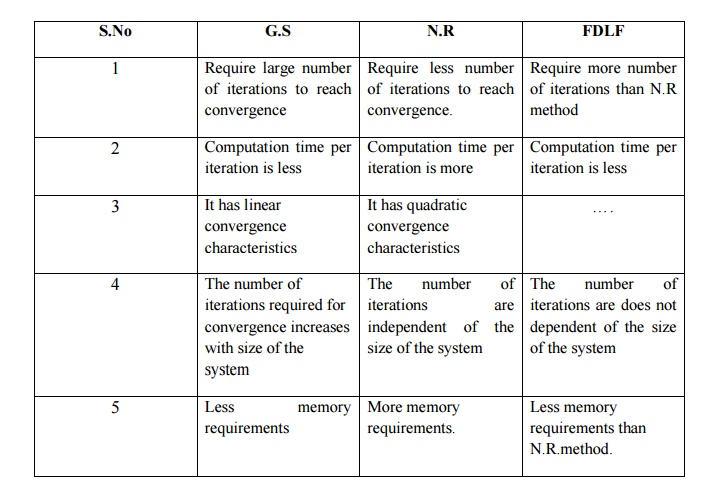Home | | Power System Analysis | Compare the Gauss Seidel and Newton Raphson Methods of Load Flow Study

# Compare the Gauss Seidel and Newton Raphson Methods of Load Flow StudyY matrix of the sample power system as shown in fig. Data for this system is given in table.

COMPARE THE GAUSS SEIDEL AND NEWTON RAPHSON METHODS OF LOAD FLOW STUDYY matrix of the sample power system as shown in fig. Data for this system is given in table.Advantages: Calculations are simple and so the programming task is lessees. The memory requirement is less. Useful for small systems;

Disadvantages: Requires large no. of iterations to reach converge .Not suitable for large systems. Convergence time increases with size of the system